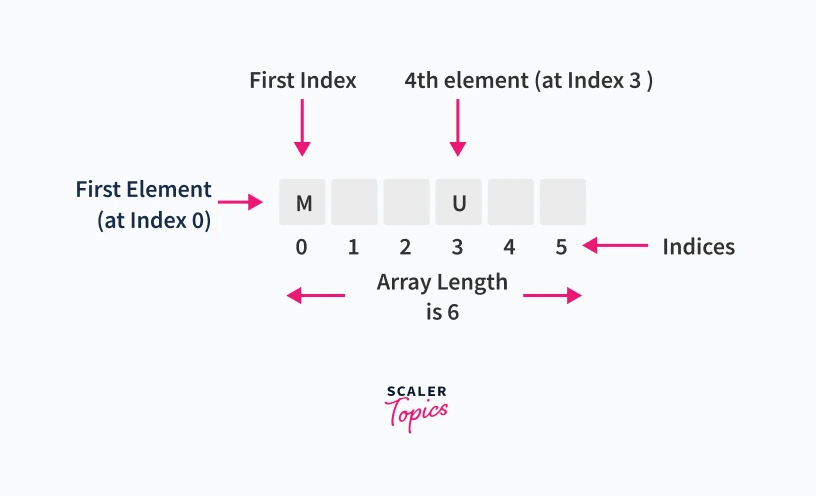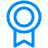# One Dimensional Array in CChallenge Inside! : Find out where you stand! Try quiz, solve problems & win rewards!

## Overview

We all know that we can use a variable to store a single value but if we have to store 100 values at a time, then declaring and initializing 100 variables is not an optimized way of doing things. For this purpose, we have arrays in C to store data of a similar data type. Arrays in C are classified into one-dimensional, two-dimensional, and multi-dimensional arrays. Array indices always start from 0 and end with size-1.

## Scope

• This article starts with introducing one-dimensional arrays in C, and we learn about array initializing, its declaration, and accessing its elements.
• We also learn about compile-time and runtime initialization and some important points to remember during array declaration in C.

## What is an Array?

An array is a collection of one or more values of the same data type stored in contiguous memory locations. The data type can be user-defined or even any other primitive data-type. Elements of an array can be accessed with the same array name by specifying the index number as the location in memory.

## Types of Arrays

Arrays in C are classified into three types:

• One-dimensional arrays
• Two-dimensional arrays
• Multi-dimensional arrays

## Introduction to One Dimensional Array in C

We can visualize a one-dimensional array in C as a single row to store the elements. All the elements are stored at contiguous memory locations. Now, we will see how to declare, initialize and access array elements:### Array Declaration

While declaring a one-dimensional array in C, the data type can be of any type, and also, we can give any name to the array, just like naming a random variable. Syntax:

``````int arr; //arr is the array name of type integer, and 5 is the size of the array
``````

### Array Initialization

In static uninitialized arrays, all the elements initially contain garbage values, but we can explicitly initialize them at their declaration.

Syntax:

``````<data_type> <arr_name> [arr_size]={value1, value2, value3,…};
``````

Here, parameterized values are constant values separated by a comma.

We can skip the writing size of the array within square brackets if we initialize array elements explicitly within the list at the time of declaration. In that case, it will pick elements list size as array size.

Example:

``````int nums = {0, 1, 2, 3, 4}; //array nums is initialized with elements 0,1,2,3,4
``````

If we want to initialize all elements of an integer array to zero, we could simply write:

``````int <array name>[size] = {0};
``````

### Array Accessing

In one-dimensional arrays in C, elements are accessed by specifying the array name and the index value within the square brackets. Array indexing starts from 0 and ends with size-1. If we try to access array elements out of the range, the compiler will not show any error message; rather, it will return some garbage value.

Syntax:

``````<arr_name>[index];
``````

Example:

``````int nums = {0, 1, 2, 3, 4};
printf("%d", nums); //Array element at index 0 is printed
printf("%d", nums[-1]); //Garbage value will be printed
``````

C Program to illustrate declaration, initialization, and accessing of elements of a one-dimensional array in C:

``````#include < stdio.h >

int main() {
//declaring and initializing one-dimensional array in C
int arr = {10, 20, 30};

// After declaration, we can also initialize the array as:
// arr = 10; arr = 20; arr = 30;

for (int i = 0; i < 3; i++) {
// accessing elements of array
printf(" Value of arr[%d]: %d\n", i, arr[i]);
}
}
``````

Output:

`````` Value of arr: 10
Value of arr: 20
Value of arr: 30
``````

In this C programming code, we have initialized an array at the time of declaration with size 3 and array name as arr. At the end of the code, we are trying to print the array values by accessing its elements.

## Rules for Declaring One Dimensional Array in C

• Before using and accessing, we must declare the array variable.
• In an array, indexing starts from 0 and ends at size-1. For example, if we have arr of size 10, then the indexing of elements ranges from 0 to 9.
• We must include data type and variable name while declaring one-dimensional arrays in C.
• We can initialize them explicitly when the declaration specifies array size within square brackets is not necessary.
• Each element of the array is stored at a contiguous memory location with a unique index number for accessing.

## Initialization of One-Dimensional Array in C

After declaration, we can initialize array elements or simply initialize them explicitly at the time of declaration. One-Dimensional arrays in C are initialized either at Compile Time or Run Time.

### Compile-Time Initialization

Compile-Time initialization is also known as static-initialization. In this, array elements are initialized when we declare the array implicitly.

Syntax:

``````<data_type> <array_name> [array_size]={list of elements};
``````

Example:

``````int nums = {0, 1, 2, 3, 4};
``````

C Program to illustrate Compile-Time Initialization:

``````#include <stdio.h>
int main(){
int nums={0,1,2};
printf(" Compile-Time Initialization Example:\n");
printf(" %d ",nums);
printf("%d ",nums);
printf("%d ",nums);
}
``````

Output:

``````0 1 2
``````

In this C program code, we have initialized an array nums of size 3 and elements as 0,1 and 2 in the list. This is compile-time initialization, and then at the end, we have printed all its values by accessing index-wise.

### Run-Time Initialization

Runtime initialization is also known as dynamic-initialization. Array elements are initialized at the runtime after successfully compiling the program.

Example:

``````scanf("%d", &nums); //initializing 0th index element at runtime dynamically
``````

C Program to illustrate Run-Time Initialization:

``````#include < stdio.h >

int main() {

int nums;
printf("\n Run-Time Initialization Example:\n");
printf("\n Enter array elements: ");

for (int i = 0; i < 5; i++) {
scanf("%d", & nums[i]);
}

printf(" Accessing array elements after dynamic Initialization: ");

for (int i = 0; i < 5; i++) {
printf("%d ", nums[i]);
}

return 0;
}
``````

Input

``````Run-Time Initialisation Example:

Enter array elements: 10 20 30 40 50
``````

Output:

``````Accessing array elements after dynamic Initialization: 10 20 30 40 50
``````

To demonstrate runtime initialization, we have just declared an array nums of size 5 in this C programming code. After that, within a loop, we ask the user to enter the array values to initialize them after compiling the code. In the end, we have printed its values by accessing them index-wise.

## Copying One-Dimensional Arrays in C

If we have two arrays - array1 and array2, one is initialized and another array is just declared, and suppose, if we have to copy array1 elements to array2 then we can't simply just write:

``````int array1 = {0, 1, 2, 3, 4};
int array2;
array2 = array1; //This statement is wrong, it will produce an error
``````

The primary condition to copy an array is that the copy array's size should be less than the original array.

Program to illustrate copying of elements of a one-dimensional array in C

``````#include < stdio.h >

int main() {
int array1 = {10, 20, 30, 40, 50};
int array2;

printf("Copying One-Dimensional Arrays in C:\n");
printf("Array1 elements: ");

for (int i = 0; i < 5; i++) {
printf("%d ", array1[i]);
array2[i] = array1[i]; // Copying array1 elements to array2
}

printf("\nArray2 elements after copying: ");

for (int i = 0; i < 5; i++) {
printf("%d ", array2[i]);
}
}
``````

Output:

``````Copying One-Dimensional Arrays in C:
Array1 elements: 10 20 30 40 50
Array2 elements after copying: 10 20 30 40 50
``````

In this C programming code, we have taken two arrays: array1 and array2. array1 has been initialized at the time of declaration, and to illustrate the concept of copying array elements, we are assigning array1 values to array2 within a loop. At the end, we printed the values of both arrays.

## Points to Remember About Array in C

• Arrays in C are a collection of similar data-type elements stored at contiguous memory locations.
• In arrays in C, all the elements have the same data type and can be accessed by their unique index value.
• Array indexing starts from 0 and ends with size-1.
• One-dimensional arrays in C can be initialized statically (during compile-time) or dynamically (during runtime).
• We must include the data type, variable name for the array, and size of the array in square brackets while declaring one-dimensional arrays in C.

## Conclusion

• Arrays in C are derived data types containing similar data-type elements.
• In one-dimensional arrays in C, indexing starts from 0 and ends at size-1, and if we try to access an element out of range, it will return garbage value.
• We must include a data type, variable name for array, and array size in square brackets while declaring one-dimensional arrays in C.
• One-dimensional arrays in C can be initialized statically (during compile-time) or dynamically (during runtime).
• All the elements of an array are stored at contiguous memory locations, and therefore, we can access them using their unique index number.
Free Courses by top Scaler instructorsCertificates
C Tutorial
This program includes modules that cover the basics to advance constructs of C Tutorial. The highly interactive and curated modules are designed to help you become a master of this language.'
If you’re a learning enthusiast, this is for you.Criteria
Upon successful completion of all the modules in the hub, you will be eligible for a certificate.